# Chapter 14 Quadrilaterals RD Sharma Solutions Exercise 14.3 Class 9 Maths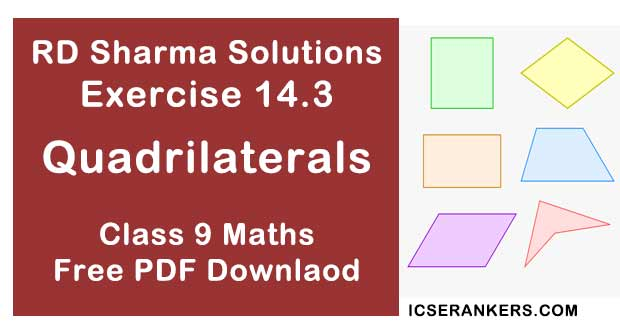Chapter Name RD Sharma Chapter 14 Quadrilaterals Exercise 14.3 Book Name RD Sharma Mathematics for Class 10 Other Exercises Exercise 14.1Exercise 14.2Exercise 14.4 Related Study NCERT Solutions for Class 10 Maths

### Exercise 14.3 Solutions

1. In a parallelogram ABCD, determine the sum of angles ∠C and ∠D.

Solution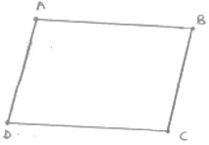∠C and ∠D are consecutive interior angles on the same side of the transversal
∴ ∠C + ∠D = 180°

2. In a parallelogram ABCD , if ∠B = 135°, determine the measures of its other angles.

Solution

Given ∠B = 135°
ABCD is a parallelogram
∴ ∠A = ∠C, ∠B = ∠D and ∠A + ∠B = 180°
∠A + ∠B = 180°
∠A = 45°
⇒ ∠A = ∠C = 45° and ∠B = ∠C = 135°

3. ABCD is a square. AC and BD intersect at O. State the measure of ∠AOB.

Solution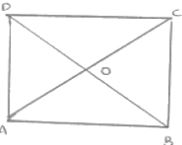Since, diagonals of square bisect each other at right angle

4. ABCD is a rectangle with ∠ABD = 40. Determine ∠DBC.

Solution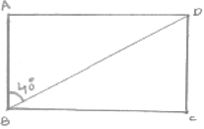We have,
∠ABC = 90°
⇒ ∠ABD + ∠DBC = 90°  [∵∠ABD = 40°]
⇒ 40 + ∠DBC = 90
∴∠DBC = 50

5. The sides AB and CD of a parallelogram ABCD are bisected at E and F. Prove that EBFD is a parallelogram.
Solution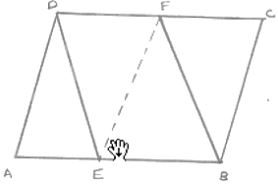Since ABCD is a parallelogram
∴ AB || DC and AB = DC
⇒ EB || DF and 1/2 AB = 1/2 DC
⇒ EB || DF  and EB = DF
EBFD is a parallelogram

6. P and Q are the points of trisection of the diagonal BD of a parallelogram ABCD. Prove that CQ is parallel to AP. Prove also that AC bisects PQ.
Solution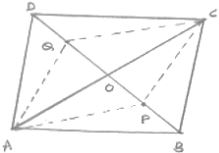We know that, diagonals of a parallelogram bisect each other
∴ OA = OC and OB = OD
Since P and Q are point of intersection of BD
∴ BP = PQ = QD
Now, OB  = OD and BP = QD
⇒ OB - BP  = OD - QD
⇒ OP = OQ
Thus in quadrilateral APCQ, we have
OA = OC and OP  = OQ
⇒ diagonals of quadrilateral APCQ bisect each other
∴ APCQ  is a parallelogram
Hence AP || CQ

7. ABCD is a square E, F, G and H are points on AB, BC, CD and DA respectively, such that AE = BF = CG = DH. Prove that EFGH is a square.
SolutionWe have,
AE = BF = CG = DH = x(say)
∴ BE = CF = DG = AH = y(say)
In Δ's AEH and BEF, we have
AE = BF
∠A = ∠B
And AH = BE
So, by SAS configuration criterion, we have
ΔAEH ≅ ΔBFE
⇒ ∠1 = ∠2 and ∠3 = ∠4
But ∠1 + ∠3 = 90° and ∠2 + ∠4 = 90°
⇒ ∠1 + ∠3 + ∠2 + ∠4 = 90° + 90°
⇒ ∠1 + ∠4 + ∠1 + ∠4 = 180°
⇒ 2(∠1 + ∠4) = 180°
⇒ ∠1 + ∠4 = 90°
⇒ ∠HEF = 180 - 90 = 90°
Similarly we have ∠F = ∠G = ∠H = 90°.
Hence, EFGH is a square

8. ABCD is a rhombus, EABF is a straight line such that EA = AB = BF. Prove that ED and FC when produced meet at right angles.
Solution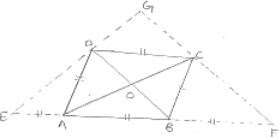We know that the diagonals of a rhombus are perpendicular bisector of each other
∴ OA = OC, OB = OD, ∠AOD = ∠COD = 90°
And ∠AOB = ∠COB = 90°
In Δ BDE, A and O are mid points of BE and BD respectively
OA || DE
OC || DG
In ΔCFA, B and O are mid points of AF and AC respectively
∴ OB || CF
OD || GC
Thus, in quadrilateral DOCG, we have
OC || DG and OD || GC
⇒ DOCG is a parallelogram
∠DGC = ∠DOC
∠DGC = 90°

9. ABCD is a parallelogram, AD is produced to E so that DE = DC and EC produced meets AB produced in F. Prove that BF = BC.
Solution
Draw a parallelogram ABCD with AC and BD intersecting at O
Produce AD to E such that DE = DC
Join EC and produce it to meet AB produced at F.
In ΔDCE,
∴ ∠DCE = ∠DEC  ...CD [In a triangle, equal sides have equal angles opposite]
AB || CD  (Opposite sides of the parallelogram are parallel)
∴ AE || CD  (AB Lies on AF)
AF || CD and EF is the transversal.
∴ ∠DCE = ∠BFC  ...(2)  [Pair of corresponding angles]
From (1) and (2), we get
∠DEC = ∠BFC
In ΔAFE,
∠AFE = ∠AEF (∠DEC = ∠BFC)
∴ AE = AF  (In a triangle, equal angles have equal sides opposite to them)
⇒ AD + DE = AB + BF
⇒ BC + AB = AB + BF  [∵ AD = BC, DE = CD and CD = AB, AB = DE]
⇒ BC = BF